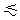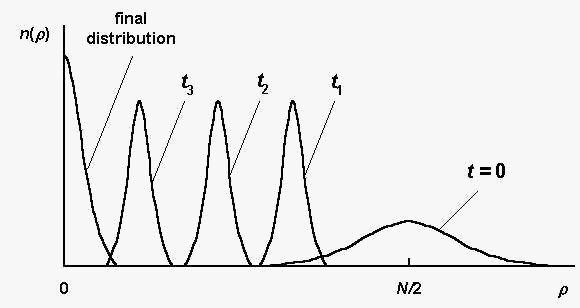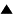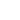# Estimation of the evolution rate

We estimate here the evolution rate for the Quasispecies model, which describes the evolution of informational sequences population {Sk} , k = 1,..., n. The sequence symbols are taken from an alphabet, containing l letters, the sequence length equals to N . The evolution process consists of consequent generations, which include selection and mutations of the sequences Sk .

We consider the stochastic case (lN >> n) under the following simplifying assumptions:
 ; 1) the alphabet consists of two letters (l = 2), namely, sequence symbols take the values: Ski = 1, -1; k = 1,..., n ; i = 1,..., N ; ; 2) the selective value of any sequence S is defined as:
 f(S) = exp[-b r(S,Sm)], (1)
 where b is a selection intensity parameter, r(S,Sm) is the Hamming distance between the given sequence S and the master sequence Sm ; 3) the mutation intensity P , that is the probability to reverse a sign of any symbol (Ski --> - Ski) during mutations, is sufficiently small:
 PNb, 1. (2)

Note, that for large P , the already found "good" sequences could be lost, so the inequality (2) is a condition for the successful evolutionary search of the sequences with large selective values (see [1,2] for details). The inequality (2) implies also a rather large selection intensity.

In addition, we assume that the population size n is sufficiently large, so a neutral selection effect  can be neglected (see below for the more detailed consideration).

Fig. 1 illustrates schematically the sequence distribution dynamics during the evolution process. Here n(r) is the number of sequences S , such that r(S,Sm) = r in a considered population; t is the generation number.Fig. 1. The sequence distribution n(r) at different generations t ; t3 > t2 > t1 (schematically, according to the computer simulations ).

The initial sequence distribution (t = 0) is a random one, it spreads in the vicinity of the value r0 = N/2 (r0 is the mean distance between an arbitrary sequence S and the master one Sm). The sequences with small r , having large selective values, are absent in the initial population. At the first several generations, the sequences, having maximal available in initial population selective value (corresponding to the left edge of the distribution at t = 0), are quickly selected, and the distribution becomes more narrow than the initial one. Such a distribution is shown in Fig.1 by the curve at t1 .

At further generations the distribution is shifted to the left (the curves at t2 , t3) until the final distribution (placed near r = 0) is reached. Because the selection intensity b is rather large (see (2)), the "shift" process is limited mainly by mutations. Typically of the order of dt = (PN)-1 generations (dt is typical time for one mutation per sequence) are needed to shift the distribution to the left on the value dr = 1. So, we can estimate the total number of evolution generations by the value
 T ~ dt x (N/2)/dr ~ (N/2)x(PN)-1. (3)

Eq. (3) characterizes roughly the evolution rate.

So far we have neglected the neutral selection effect, which is essential at a small population size . The neutral selection is the random fixation in a population an arbitrary "species", regardless of a selective value. It could suppress the search of the "good" sequences. Typical time Tn of neutral selection is of the order of a population size n (see Neutral evolution game for details). We can neglect the neutral selection, if the total generation number T is smaller than Tn :
 T < Tn ~ n . (4)

The inequality (4) is a condition, at which the estimation of the evolution rate (3) is valid.

In addition, we can construct the "optimal" evolution process, which involves the minimal total number of participants ntotal = nT under condition that master sequence is found. The "optimal" evolution corresponds to the maximal permissible mutation intensity P ~ N -1 (see (2)). At this P , according to (3), we have T ~ (N/2) . Taking into account (4), we can set n ~ 2N and obtain finally:
 ntotal = nT ~ N 2 (5)

The estimation (5) characterizes the effectiveness of the evolution process as an algorithm for search of the optimal (master) sequence.

The estimations (3), (5) were confirmed by computer simulations .

References:

1. M.Eigen, P.Schuster. "The hypercycle: A principle of natural self-organization". Springer Verlag: Berlin etc. 1979.

2. V.G.Red'ko. Biofizika. 1986. Vol. 31. N.3. P. 511. V.G.Red'ko. Biofizika. 1990. Vol. 35. N.5. P. 831 (In Russian).

3. M. Kimura. "The neutral theory of molecular evolution". Cambridge Un-ty Press. 1983.

 HomeMetasystem Transition TheoryEvolutionary TheoryMathematical Modeling of EvolutionModels of molecular-genetic systems originQuasispecies Up Prev.Next Down